## Introduction

Decision Tree Learning is a supervised Machine Learning algorithm that is used extensively for classification and regression problems. A decision tree model is like the non-linear data structure tree, but its nodes are created by understanding and inferring rules given in the dataset. Decision trees predict the value of a target variable by implementing the rules it has learned from the featured data in the dataset as it traverses along the nodes to reach a leaf node.Decision trees can be built using several mathematical methods, some of them are:

1) ID3 Algorithm: Using this method, decision trees are built iteratively by finding out the maximum Information Gain among all the featured data columns to be represented as the node of the tree.

2) C4.5 Algorithm: It is an extension of the ID3 Algorithm where Gain Ratio is inferred from the Information Gain and Split Ratio to be the node splitting factor.

3) J48 Algorithm: It is the open-source commercialized version of the C4.8 algorithm (which is a modification of C4.5 algorithm in terms of algorithmic optimization). J48 algorithm can consider data with null values into the prediction model, using the same mathematical method as C4.5 algorithm.

4) CART Algorithm: A decision tree using CART Algorithm is made using the concept of Gini Index. For each iteration, column having the least Gini Impurity will be considered as the node for the decision tree.

## ID3 Algorithm

Iterative Dichotomiser 3 algorithm builds a decision on two metrics: Entropy and Information Gain of each featured data column. Entropy is the measure of uncertainty of a label in a particular column, whereas Information Gain is the measure of feature information of a column in the dataset.As we find these information for each column in the dataset, we can easily find the entropy. Information gain will be the difference between entropy before and after the split takes place.In order to find the nodes for each level of the decision tree, we need to iteratively calculate the entropy of each column label, followed by the information gain of the entire column.

Here are the steps to find the nodes of the decision tree:

1) Find the entropy of before split

2) For each column, find entropy of each label

3) For each column, find the information gain.

4) Column becomes the node of the tree if it has the highest information gain.

We will understand the concept in detail by using an example.

Consider the dataset below where “Covid” is the label of interest: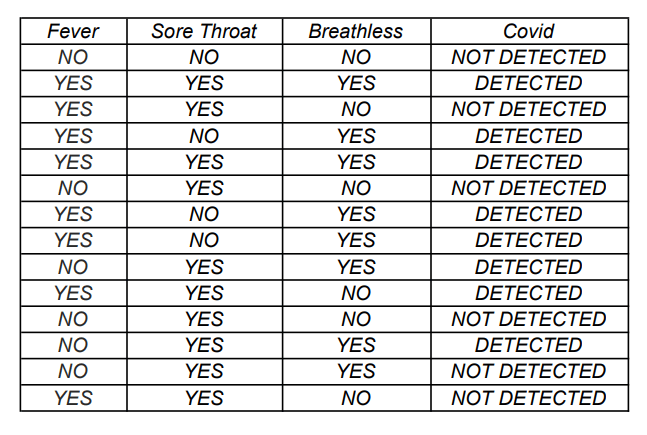Let us build the decision tree using the above dataset. We assume ‘Detected’ as positive and ‘Not Detected’ as negative. All logarithms are calculated with the base as 2.

Here, n = 14, p = 8, q = 6

Therefore, Entropy(target) = – (8*log(8/14)/14) – (6*log(6/14)/14) = 0.99

Considering the column “Fever”:

Total items with label ‘Yes’ = 8

Total items with label ‘No’ = 6

Positive correspondences for label ‘Yes’ = 6

Positive correspondences for label ‘No’ = 2

Negative correspondences of label ‘Yes’ = 2

Negative correspondences of label ‘No’ = 4

Entropy(Yes) = – (6*log(6/8)/8) – (2*log(2/8)/8) = 0.81

Entropy(No) = -(2*log(2/6)/6) – (4*log(4/6)/6) = 0.91

Information Gain(Fever) = 0.99 – ((8*0.81)/14 + (6*0.91)/14) = 0.13We repeat this process for the other columns in the dataset as well. The insights of each column is given below: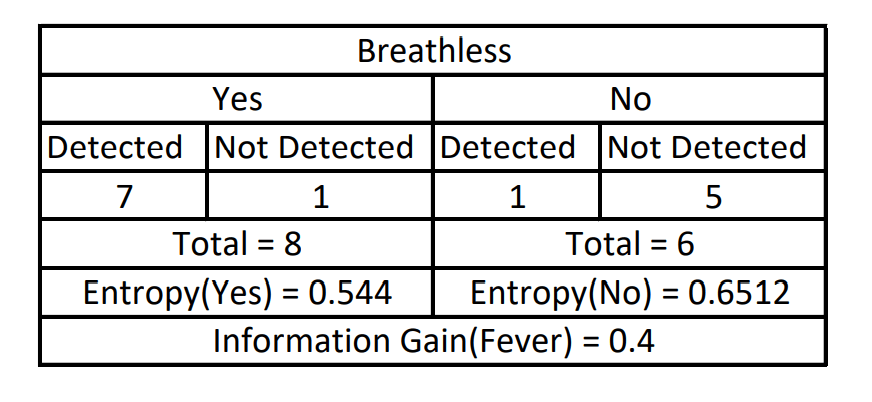The highest information gain calculated belongs to the column “Breathless”, which becomes the root node of the Decision Tree. The root node.We follow the same procedure again. Since the current decision tree now points to two directions, it is clear that one column will become a node to each side of the root node. We begin with finding the left child node of the tree. There are two possible columns now that can be used as the node, i.e., Fever and Sore Throat. Our items of interest will be only those rows that have ‘Yes’ in Breathless column.Since the column Fever has higher gain, we make it as the left child of the Decision Tree. The process of calculation now repeats again for the right child of the root node.Please note that the column Fever can still be considered as the right child of the root node. We now consider only those dataset items that has Breathless value as ‘No’.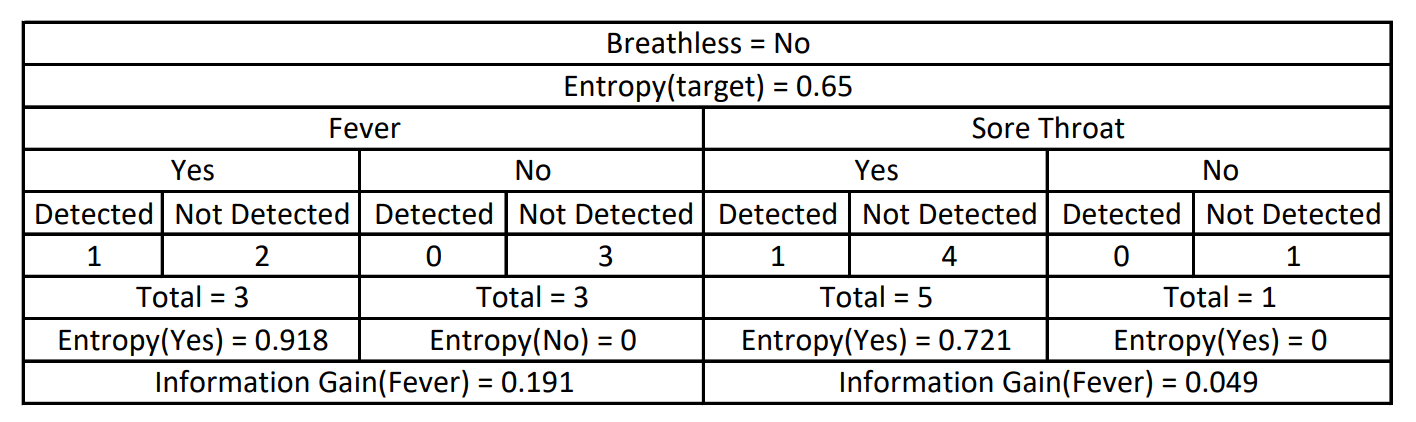Here also we find the gain of Fever column to be higher than the Sore Throat, so we make Fever as the right child.Now if we observe carefully, it can be noted that when we go for the next iteration and try to find left child of Fever (Breathless = Yes and Fever = Yes), we get pure data, which means that all the data items correspond to the label ‘Detected’ only in the label of interest. Hence, we get a leaf node, from which we can infer a prediction. The same can be said for Breathless = No and Fever = No. Refer to the images above for better understanding.Since there is only one column left, both the remaining child nodes have to filled with the column Sore Throat only.From the dataset, we see that there does not exist Sore Throat = No in both the cases of the decision tree. Therefore the only label we have is Sore Throat = Yes. In this scenario, we cannot obtain a pure node, so we go for the majority.

In the case of Breathless = Yes and Fever = No, the majority corresponds to Covid = Detected. Hence we assume this answer as the leaf node.

In the case of Breathless = No and Fever = Yes, the majority corresponds to Covid = Detected. Hence we assume this answer as the leaf node.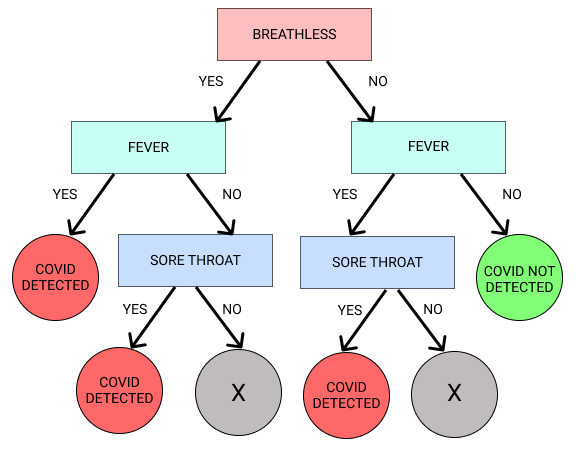Since Sore Throat = No has no value in the decision tree, we infer that if leaf node is not reached in the 2nd level of the tree, then Sore Throat will automatically be considered as Yes. Therefore, we remove the condition of Sore Throat altogether and directly write the leaf node.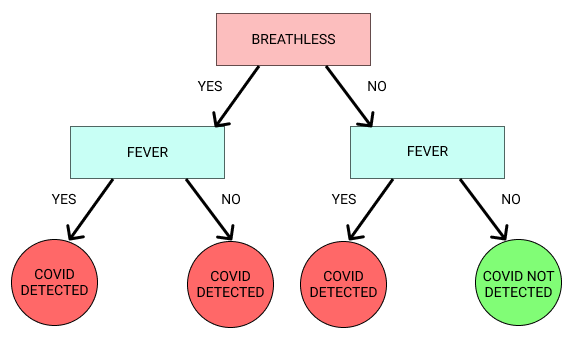This becomes our final decision tree. Let us now understand the code for decision tree classifier. There can be one further assumption in this decision tree. Whenever Breathless = Yes, we are inferring Covid = Detected only. Therefore there is no need to consider the status of Fever column. However, this pruning is not supported in ID3 algorithm because Breathless = Yes is not pure. This can be considered to be one of the drawbacks of the ID3 algorithm.

### Implementation

Let us now learn how to create a program to build a decision tree. The weka library for Kotlin had an in-built ID3 algorithm class for creating a Machine Learning model and using it for prediction. However, this class has been deprecated due to the pruning issues of ID3 that we have already discussed. Therefore, we use the upgraded version of the ID3 algorithm, called the J48 algorithm which considers pruning as an option (this algorithm prunes the tree if needed). Let us have a training dataset and a testing dataset to build an ML model and find out its prediction accuracy. We consider the following test and train data on the iris dataset.

				
@file:Repository("https://mvnrepository.com/artifact/nz.ac.waikato.cms.weka/weka-stable")
@file:DependsOn("nz.ac.waikato.cms.weka:weka-stable:3.8.0")


				
import weka.core.Instances
import weka.classifiers.Classifier
import weka.classifiers.Evaluation
import weka.core.converters.ConverterUtils


				
val sourcetrain = ConverterUtils.DataSource("train_data.arff")
val traindataset = sourcetrain.dataSet
traindataset.setClassIndex(traindataset.numAttributes() - 1)


				
val sourcetest = ConverterUtils.DataSource("test_data.arff")
val testdataset = sourcetest.dataSet
testdataset.setClassIndex(testdataset.numAttributes() - 1)


				
import weka.classifiers.trees.J48
println("----J48----")
val dtj48 = J48()
dtj48.buildClassifier(traindataset)
println(dtj48.getCapabilities().toString())



----J48----
Capabilities: [Nominal attributes, Binary attributes, Unary attributes, Empty nominal attributes, Numeric attributes, Date attributes, Missing values, Nominal class, Binary class, Missing class values]
Dependencies: []
min # Instance: 0
				
println(dtj48.graph())


digraph J48Tree {
N0 [label="petalwidth" ]
N0->N1 [label="<= 0.6"]
N1 [label="Iris-setosa (50.0)" shape=box style=filled ]
N0->N2 [label="> 0.6"]
N2 [label="petalwidth" ]
N2->N3 [label="<= 1.7"]
N3 [label="petallength" ]
N3->N4 [label="<= 4.9"]
N4 [label="Iris-versicolor (48.0/1.0)" shape=box style=filled ]
N3->N5 [label="> 4.9"]
N5 [label="petalwidth" ]
N5->N6 [label="<= 1.5"]
N6 [label="Iris-virginica (3.0)" shape=box style=filled ]
N5->N7 [label="> 1.5"]
N7 [label="Iris-versicolor (3.0/1.0)" shape=box style=filled ]
N2->N8 [label="> 1.7"]
N8 [label="Iris-virginica (46.0/1.0)" shape=box style=filled ]
}
				
val evalj48 = Evaluation(traindataset)
evalj48.evaluateModel(dtj48, testdataset)
System.out.printf("Correct: %.2f\n",evalj48.pctCorrect())
System.out.printf("Incorrect: %.2f\n",evalj48.pctIncorrect())
println(evalj48.toMatrixString())
System.out.printf("Precision: %.2f\n", evalj48.precision(1)*100)
System.out.printf("Recall: %.2f\n", evalj48.recall(1)*100)
System.out.printf("F1 score: %.2f\n", evalj48.fMeasure(1)*100)



Let us compare the performance of our tree with some of the other Decision Tree algorithms. We will compare J48 with REP Tree and LMT.

#### REP Tree

Reduced Error Pruning Tree is an extension of the C4.5 algorithm wherein there is an attempt made by the algorithm to reduce a subtree into a single node without reducing the accuracy of the tree.

				
import weka.classifiers.trees.REPTree
println("----REP----")
val dtrep = REPTree()
dtrep.buildClassifier(traindataset)
println(dtrep.getCapabilities().toString())


----REP----
Capabilities: [Nominal attributes, Binary attributes, Unary attributes, Empty nominal attributes, Numeric attributes, Date attributes, Missing values, Nominal class, Binary class, Numeric class, Date class, Missing class values]
Dependencies: []
min # Instance: 1
				
println(dtrep.graph())


digraph Tree {
edge [style=bold]
N58b112d4 [label="1: petallength"]
N58b112d4->N1b866a69 [label=" < 2.5"]
N1b866a69 [label="2 : Iris-setosa (33/0) [17/0]"shape=box]
N58b112d4->N16c9ee96 [label=" >= 2.5"]
N16c9ee96 [label="3: petalwidth"]
N16c9ee96->N385b9f49 [label=" < 1.75"]
N385b9f49 [label="4 : Iris-versicolor (36/3) [18/2]"shape=box]
N16c9ee96->N682c0d24 [label=" >= 1.75"]
N682c0d24 [label="5 : Iris-virginica (31/1) [15/0]"shape=box]

}
				
val evalrep = Evaluation(traindataset)
evalrep.evaluateModel(dtrep, testdataset)
System.out.printf("Correct: %.2f\n",evalrep.pctCorrect())
System.out.printf("Incorrect: %.2f\n",evalrep.pctIncorrect())
println(evalj48.toMatrixString())
System.out.printf("Precision: %.2f\n", evalrep.precision(1)*100)
System.out.printf("Recall: %.2f\n", evalrep.recall(1)*100)
System.out.printf("F1 score: %.2f\n", evalrep.fMeasure(1)*100)



#### LMT

Logistic Model Tree is an algorithm that combines the Logistic Regression concept and Decision Trees concept. An LR model is brought in by every node but the splitting criteria are the same as the C4.5 algorithm.

				
import weka.classifiers.trees.LMT
println("----LMT----")
val dtlmt = LMT()
dtlmt.buildClassifier(traindataset)
println(dtlmt.getCapabilities().toString())


----LMT----
Capabilities: [Nominal attributes, Binary attributes, Unary attributes, Empty nominal attributes, Numeric attributes, Date attributes, Missing values, Nominal class, Binary class, Missing class values]
Dependencies: []
min # Instance: 1
				
println(dtlmt.graph())


digraph LMTree {
N0 [label="LM_1:18/18 (150)" shape=box style=filled]
}
				
val evallmt = Evaluation(traindataset)
evallmt.evaluateModel(dtlmt, testdataset)
System.out.printf("Correct: %.2f\n",evallmt.pctCorrect())
System.out.printf("Incorrect: %.2f\n",evallmt.pctIncorrect())
println(evalj48.toMatrixString())
System.out.printf("Precision: %.2f\n", evallmt.precision(1)*100)
System.out.printf("Recall: %.2f\n", evallmt.recall(1)*100)
System.out.printf("F1 score: %.2f\n", evallmt.fMeasure(1)*100)



#### Conclusion

From the F1 Scores inferred after fitting the 3 DTs, it can be concluded that the most efficient model for the testing dataset is LMT, which correctly predicts all the data items. This is followed by J48 Tree (96.97%) and then REP Tree (94.12%).